# Band Math results not has expected and divided by zero error

Dear Step Community,
I’m trying “simple” band math expression for aCDOM(420nm): 5.13*(B3/B4)^(-2.67)
and outcome isn’t pretty, “SNAP ERROR: java exception /by zero”.

The curious part is: If I create a new band (for instance newBi) has newBi=B3/B4 I obtain a result (image) with the following min and max values (0.3507,21.1111):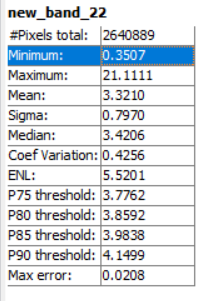If I then apply the following exponent to the previous band image (newBi)^ (-2.67) you get the following min and max values (-21,-1)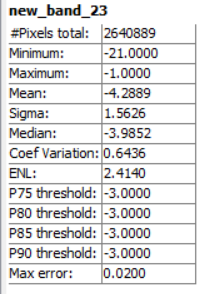which is not true because if you apply (0.3507)^(-2.67) you get 16.41 and (21.11)^(-2.67) you get 290.8E-06. Very strange.

If you compute the statistics for B3:And B4: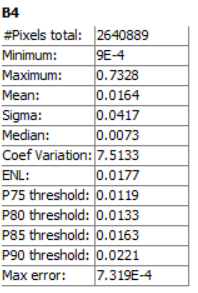The thing is that we should at least get the correct numbers for the (newBi)^(-2.67) and that isn’t happening.

I don’t know if could explain the problem and if it makes in sense to take a look at it.
I would like to compute this expression in band math, but I’m having a really hard time with the divide by zero error java arithmetic exception.

Thank you for the understanding

I recall another instance of unexpected results (using BEAM or NASA SeaDAS 7, so years ago). Bandmath was being applied to raw unscaled data rather than the geophysical values (e.g., after scaling had been applied).

I say something is not right with SNAP band math. I processed this two band raster calculation with S2A B3 and B4 in R studio and take a look at the results:

Blockquote
class : RasterLayer
dimensions : 3140, 1256, 3943840 (nrow, ncol, ncell)
resolution : 10, 10 (x, y)
extent : 511050, 523610, 4568580, 4599980 (xmin, xmax, ymin, ymax)
crs : +proj=utm +zone=29 +datum=WGS84 +units=m +no_defs +ellps=WGS84 +towgs84=0,0,0
source : memory
names : layer
values : 0.00149167, 2346.464 (min, max)

From that processed result it was fairly simple to attribute a color map on QGis:

The same formula was applied to a Landsat image in R, but again SNAP band math failed. Take a look to R processed result:

Blockquote
class : RasterLayer
dimensions : 1048, 419, 439112 (nrow, ncol, ncell)
resolution : 30, 30 (x, y)
extent : 511035, 523605, 4568565, 4600005 (xmin, xmax, ymin, ymax)
crs : +proj=utm +zone=29 +datum=WGS84 +units=m +no_defs +ellps=WGS84 +towgs84=0,0,0
source : memory
names : layer
values : 0.1380741, 21.33981 (min, max)

Eventually something isn’t coming up ok in SNAP BMaths

Are you aware that SNAP applies a scaling factors to many products while r works on the raw image data?
Some considerations here: About scaling factor and exporting product etc

Calculations performed in SNAP might give different results than based on the rasters alone.

Hi ABraun,
Thank you for the reply. I don’t know if I really understand what you are trying to explain to me.
But let me share this numbers. I think I’m using (in the case of R processing box) the correct values for the images, for instance, for a Sentinel 2 MS image, take a look:

Additionally, a brief summary of the pixel values for the Bands processed in R, after export from SNAP:

Blockquote
Aerosols Blue Green Red RE1 RE2 RE3 NIR narrow_NIR SWIR1 SWIR2
Min. 0.0030 0.0000 0.0000 0.0000 0.0000 0.0000 0.0000 0.0000 0.0000 0.0000 0.0000
1st Qu. 0.0252 0.0295 0.0210 0.0062 0.0036 0.0017 0.0018 0.0019 0.0007 0.0001 0.0001
Median 0.0286 0.0395 0.0349 0.0116 0.0069 0.0029 0.0031 0.0030 0.0017 0.0001 0.0003
3rd Qu. 0.0363 0.0488 0.0593 0.0543 0.0917 0.1435 0.1659 0.1666 0.1830 0.1635 0.1071
Max. 0.4922 0.6544 0.7208 0.8320 0.6638 0.6489 0.7022 0.8164 0.7580 1.2114 1.1901
NA’s 0.0000 0.0000 0.0000 0.0000 0.0000 0.0000 0.0000 0.0000 0.0000 0.0000 0.0000

Besides how can we have in SNAP BandMath the following results:
B3/B4 --> (min, max) = (0.3507,21.1111) and then you apply in exponent (0.3507,21.1111)^(-2.67) and you get min and max values -21 and -1? You should get at least, for those values 290.8E-06. and 16.41.

Moreover the BandMath produces a new Band image for the ratio B3/B4, but it doesn’t produce for the same ratio to the exponent (B3/B4)^(-2.67).

Just a ending note:
In fact for the Landsat 8 image the pixel numbers are in DN, here is brief summary:

Blockquote
Aerosols Blue Green Red NIR SWIR1 SWIR2
Min. -2000 -1821 -355 -371 -75 -4 -7
1st Qu. 371 333 223 81 21 8 4
Median 422 425 360 140 33 16 10
3rd Qu. 467 500 596 569 1819 1523 917
Max. 4770 5236 5811 5743 7135 9991 9640
NA’s 0 0 0 0 0 0 0

the min and max values are quite odd (-1.0000 and -21.0000) so they could rather be a processing artefact of invalid pixels (NoData pixels) instead of the actual minimum after the calculation.

Have you checked one specific pixel before and after calculation? This should be more reliable than the overall statistics.

Similar as @gnwiii I was referring to the scaling of some data in SNAP

Accordingly, a value of 0.4 in SNAP corresponds to a actual pixel value of 4000 in the physical raster (as maybe addressed by R)

I generated a new band (new_band1) has the result of B3/B4 with the following values for a pixel:

Info for the band ratio:

Now applying the previous band ratio the following exponent (new_band1)^(-2.67)
we get -3 value for the same pixel, when we should get (3.45)^(-2.67) = 39.8E-03:

here is the band info:

The question remains:
How can the Band Math produce a new Band image for the ratio B3/B4, but it doesn’t produce for the same ratio to the exponent (B3/B4)^(-2.67).
Because if I try to create the newband image directly (B3/B4)^(-2.67):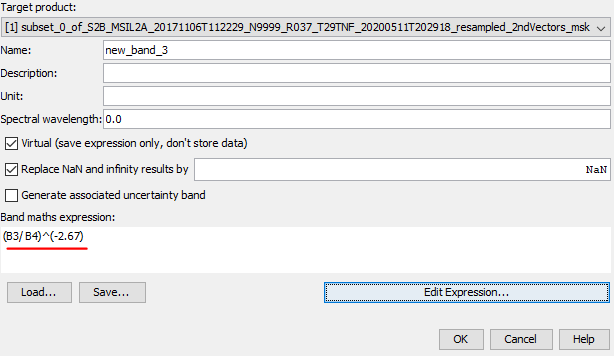you get error message: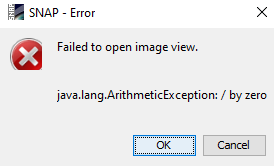Note that the base image bands are in principle ok, for instance B4

could it be that the valid pixel expression is causing troubles?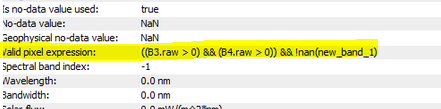It would be worth a try to remove it before the calculation.

I cannot imagine that there is a bug for such a calculation. I would write it the same actually.

well:The result is pretty much the same:

I don’t know whats wrong …

I’m also out of ideas, sorry.

ok, ABraun. Thank you for trying.

It is always dangerous trying to use multiple languages. I finally looked at the documentation for “Band Maths Expression Editor” and discovered that the “hat” operator is “bitwise XOR”. Use `pow(X,Y)`

2 Likes

good one @gnwiii!I carelessly assumed that ^ is universal, but you are probably right.

Amazing gnwiiit worked !! Thank you a lotJust a quick result:

You can also use ** instead of ^

1 Like